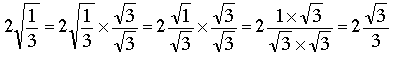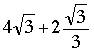SEARCH HOMEMath Central Quandaries & QueriesQuestion from Michelle, a student: How do you solve 4√3 + 2√1/3? Please show step by step.Hi Michelle,

My suggestion is that to simplify this expression you first rationalize the denominator of the second term by multiplying the numerator and denominator by √3. The second term then becomes.

Hence your expression can be written.

Find a common denominator and simplify,
PennyMath Central is supported by the University of Regina and The Pacific Institute for the Mathematical Sciences.